# NCERT Solution (Ex-3.2) - Chapter 3: Understanding Quadrilaterals, Maths, Class 8 Notes - Class 8

## Class 8: NCERT Solution (Ex-3.2) - Chapter 3: Understanding Quadrilaterals, Maths, Class 8 Notes - Class 8

The document NCERT Solution (Ex-3.2) - Chapter 3: Understanding Quadrilaterals, Maths, Class 8 Notes - Class 8 is a part of Class 8 category.
All you need of Class 8 at this link: Class 8

Exercise 3.2

Question 1: Find x in the following figures: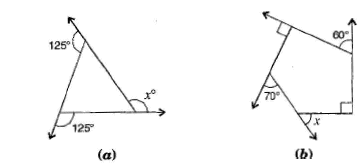(a) Here, 125o+m=180o   [Linear pair]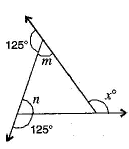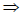m=180o-125o = 55o
and 125o+n =180o           [Linear pair]n =180o-125o = 55o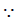Exterior angle xo = Sum of opposite interior angles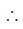xo = 55o+55o =110o

(b) Sum of angles of a pentagon = (n - 2)x180o
= (5 - 2)x180o
= 3x180o = 540o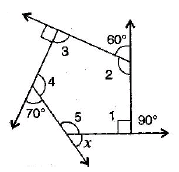By linear pairs of angles,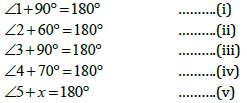Adding eq. (i), (ii), (iii), (iv) and (v),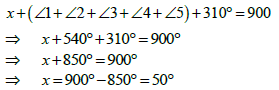Question 2:
Find the measure of each exterior angle of a regular polygon of:
(a) 9 sides

(b) 15 sides

(i) Sum of angles of a regular polygon = (n-2)x180o
= (9-2)x180o = 7x180o =1260o

Each interior angle= Sum of interior angles / Number of sides =1260o/9 =140o

Each exterior angle = 180o-140o = 40o

(ii) Sum of exterior angles of a regular polygon = 360o
Each interior angle = Sum of interior angles/Number of sides = 360o/15 =24o

Question 3:
How many sides does a regular polygon have, if the measure of an exterior angle is 24o?

Let number of sides be n.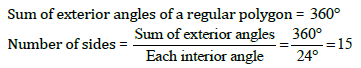Hence, the regular polygon has 15 sides.

Question 4:
How many sides does a regular polygon have if each of its interior angles is 165?

Let number of sides be n.
Exterior angle = 180o-165o=15o
Sum of exterior angles of a regular polygon = 360o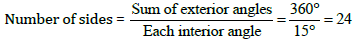Hence, the regular polygon has 24 sides.

Question 5:
(a) Is it possible to have a regular polygon with of each exterior angle as 22
o?
(b) Can it be an interior angle of a regular polygon? Why?

(a) No. (Since 22 is not a divisor of 360o )
(b) No, (Because each exterior angle is 180o - 22o =158o, which is not a divisor of 360o )

Question 6:
(a) What is the minimum interior angle possible for a regular polygon? Why?
(b) What is the maximum exterior angle possible for a regular polygon?

(a) The equilateral triangle being a regular polygon of 3 sides has the least measure of an interior angle of 60o .Sum of all the angles of a triangle = 180ox+x+x= 1803x=1800x=60o

(b) By (a), we can observe that the greatest exterior angle is 1800 - 600 =1200.

The document NCERT Solution (Ex-3.2) - Chapter 3: Understanding Quadrilaterals, Maths, Class 8 Notes - Class 8 is a part of Class 8 category.
All you need of Class 8 at this link: Class 8Use Code STAYHOME200 and get INR 200 additional OFF

Track your progress, build streaks, highlight & save important lessons and more!

,

,

,

,

,

,

,

,

,

,

,

,

,

,

,

,

,

,

,

,

,

,

,

,

,

,

,

;